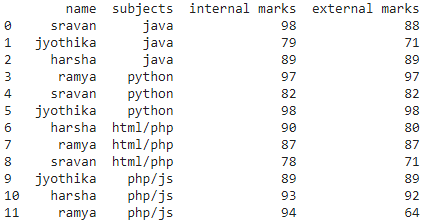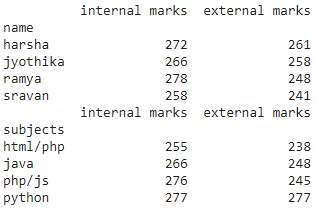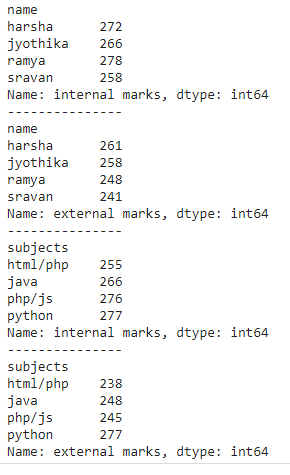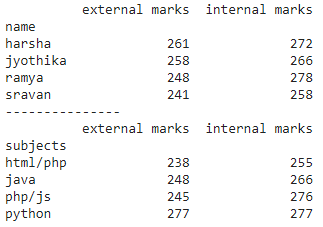Open in App
Not now

# How to Perform a SUMIF Function in Pandas?

• Last Updated : 22 Nov, 2021

sumif() function is used to perform sum operation by a group of items in the dataframe, It can be applied on single and multiple columns and we can also use this function with groupby function.

## Method 1: SUMIF on all columns with groupby()

This function is used to display sum of all columns with respect to grouped column

Syntax: dataframe.groupby(‘group_column’).sum()

where

• dataframe is the input dataframe
• group_column is the column in dataframe to be grouped
• sum() function is to perform the sum operation

## Python3

 `# import pandas module ` `import` `pandas as pd ` ` `  `# create dataframe with 4 columns ` `data ``=` `pd.DataFrame({ ` ` `  `    ``"name"``: [``'sravan'``, ``'jyothika'``, ``'harsha'``,  ` `             ``'ramya'``, ``'sravan'``, ``'jyothika'``,  ` `             ``'harsha'``, ``'ramya'``, ``'sravan'``, ``'jyothika'``, ` `             ``'harsha'``, ``'ramya'``], ` `    ``"subjects"``: [``'java'``, ``'java'``, ``'java'``, ``'python'``, ` `                 ``'python'``, ``'python'``, ``'html/php'``,  ` `                 ``'html/php'``, ``'html/php'``, ``'php/js'``, ` `                 ``'php/js'``, ``'php/js'``], ` `    ``"internal marks"``: [``98``, ``79``, ``89``, ``97``, ``82``, ``98``, ``90``, ` `                       ``87``, ``78``, ``89``, ``93``, ``94``], ` `    ``"external marks"``: [``88``, ``71``, ``89``, ``97``, ``82``, ``98``, ``80``, ` `                       ``87``, ``71``, ``89``, ``92``, ``64``], ` `}) ` ` `  `# display dataframe ` `print``(data) `

Output:## Python3

 `# import pandas module ` `import` `pandas as pd ` ` `  `# create dataframe with 4 columns ` `data ``=` `pd.DataFrame({ ` ` `  `    ``"name"``: [``'sravan'``, ``'jyothika'``, ``'harsha'``, ``'ramya'``, ` `             ``'sravan'``, ``'jyothika'``, ``'harsha'``, ``'ramya'``, ` `             ``'sravan'``, ``'jyothika'``, ``'harsha'``, ``'ramya'``], ` `    ``"subjects"``: [``'java'``, ``'java'``, ``'java'``, ``'python'``, ` `                 ``'python'``, ``'python'``, ``'html/php'``, ` `                 ``'html/php'``, ``'html/php'``, ``'php/js'``, ` `                 ``'php/js'``, ``'php/js'``], ` `    ``"internal marks"``: [``98``, ``79``, ``89``, ``97``, ``82``, ``98``, ``90``, ` `                       ``87``, ``78``, ``89``, ``93``, ``94``], ` `    ``"external marks"``: [``88``, ``71``, ``89``, ``97``, ``82``, ``98``, ``80``, ` `                       ``87``, ``71``, ``89``, ``92``, ``64``], ` `}) ` ` `  `# find sum of all columns group by name ` `print``(data.groupby(``'name'``).``sum``()) ` ` `  ` `  `# find sum of all columns group by subjects ` `print``(data.groupby(``'subjects'``).``sum``()) `

Output:## Method 2: SUMIF Function on One Column

Here we are performing sumif operation on one particular column by grouping it with one column

Syntax: dataframe.groupby(‘group_column’)[‘column_name].sum()

where

• dataframe is the input dataframe
• group_column is the column in dataframe to be grouped
• column_name is to get sum of this column with respect to grouped column
• sum() function is to perform the sum operation

## Python3

 `# import pandas module ` `import` `pandas as pd ` ` `  `# create dataframe with 4 columns ` `data ``=` `pd.DataFrame({ ` ` `  `    ``"name"``: [``'sravan'``, ``'jyothika'``, ``'harsha'``, ``'ramya'``, ` `             ``'sravan'``, ``'jyothika'``, ``'harsha'``, ``'ramya'``,  ` `             ``'sravan'``, ``'jyothika'``, ``'harsha'``, ``'ramya'``], ` `    ``"subjects"``: [``'java'``, ``'java'``, ``'java'``, ``'python'``, ` `                 ``'python'``, ``'python'``, ``'html/php'``,  ` `                 ``'html/php'``, ``'html/php'``, ``'php/js'``, ` `                 ``'php/js'``, ``'php/js'``], ` `    ``"internal marks"``: [``98``, ``79``, ``89``, ``97``, ``82``, ``98``, ``90``, ` `                       ``87``, ``78``, ``89``, ``93``, ``94``], ` `    ``"external marks"``: [``88``, ``71``, ``89``, ``97``, ``82``, ``98``, ``80``, ` `                       ``87``, ``71``, ``89``, ``92``, ``64``], ` `}) ` ` `  `# find sum of  columns group by ` `# name with internal marks column ` `print``(data.groupby(``'name'``)[``'internal marks'``].``sum``()) ` ` `  `print``(``"---------------"``) ` ` `  `# find sum of  columns group by ` `# name with external marks column ` `print``(data.groupby(``'name'``)[``'external marks'``].``sum``()) ` ` `  `print``(``"---------------"``) ` ` `  `# find sum of  columns group by ` `# subjects with internal marks column ` `print``(data.groupby(``'subjects'``)[``'internal marks'``].``sum``()) ` ` `  `print``(``"---------------"``) ` ` `  `# find sum of  columns group by ` `# subjects with external marks column ` `print``(data.groupby(``'subjects'``)[``'external marks'``].``sum``()) `

Output:## Method 3: SUMIF Operation on multiple columns

Here we will use sumif operation on multiple columns.

Syntax: dataframe.groupby(‘group_column’)[[‘column_names’]].sum()

where,

• dataframe is the input dataframe
• group_column is the column in dataframe to be grouped
• column_names are to get sum of these columns with respect to grouped column
• sum() function is to perform the sum operation

## Python3

 `# import pandas module ` `import` `pandas as pd ` ` `  `# create dataframe with 4 columns ` `data ``=` `pd.DataFrame({ ` ` `  `    ``"name"``: [``'sravan'``, ``'jyothika'``, ``'harsha'``, ``'ramya'``, ` `             ``'sravan'``, ``'jyothika'``, ``'harsha'``, ``'ramya'``,  ` `             ``'sravan'``, ``'jyothika'``, ``'harsha'``, ``'ramya'``], ` `    ``"subjects"``: [``'java'``, ``'java'``, ``'java'``, ``'python'``, ` `                 ``'python'``, ``'python'``, ``'html/php'``,  ` `                 ``'html/php'``, ``'html/php'``, ``'php/js'``,  ` `                 ``'php/js'``, ``'php/js'``], ` `    ``"internal marks"``: [``98``, ``79``, ``89``, ``97``, ``82``, ``98``, ``90``, ` `                       ``87``, ``78``, ``89``, ``93``, ``94``], ` `    ``"external marks"``: [``88``, ``71``, ``89``, ``97``, ``82``, ``98``, ``80``, ` `                       ``87``, ``71``, ``89``, ``92``, ``64``], ` `}) ` ` `  `# find sum of  columns group by name with ` `# external marks and internal marks column ` `print``(data.groupby(``'name'``)[[``'external marks'``, ` `                            ``'internal marks'``]].``sum``()) ` ` `  `print``(``"---------------"``) ` ` `  `# find sum of  columns group by subjects ` `# with external marks and internal marks column ` `print``(data.groupby(``'subjects'``)[[``'external marks'``, ` `                                ``'internal marks'``]].``sum``()) `

Output:My Personal Notes arrow_drop_up
Related Articles